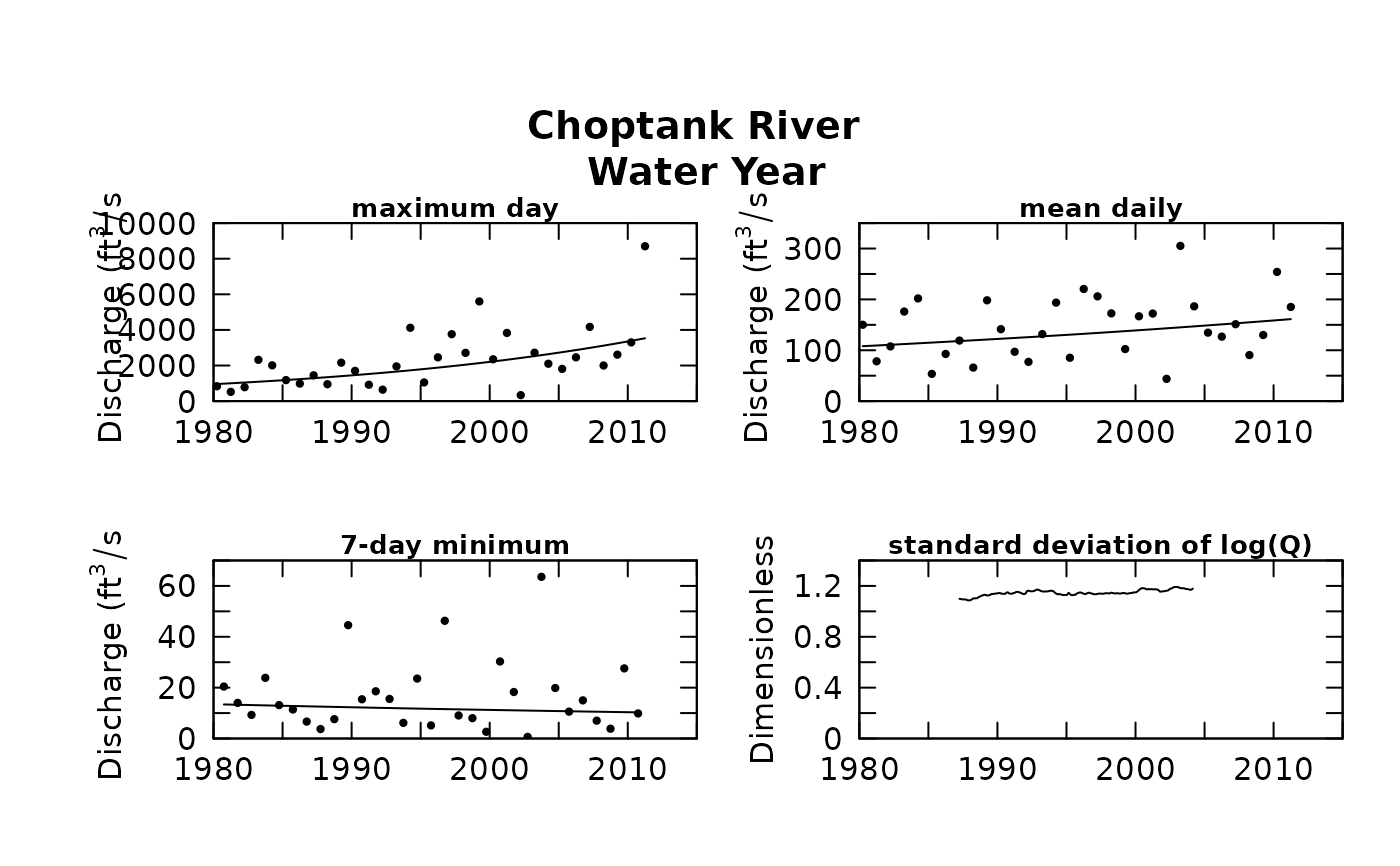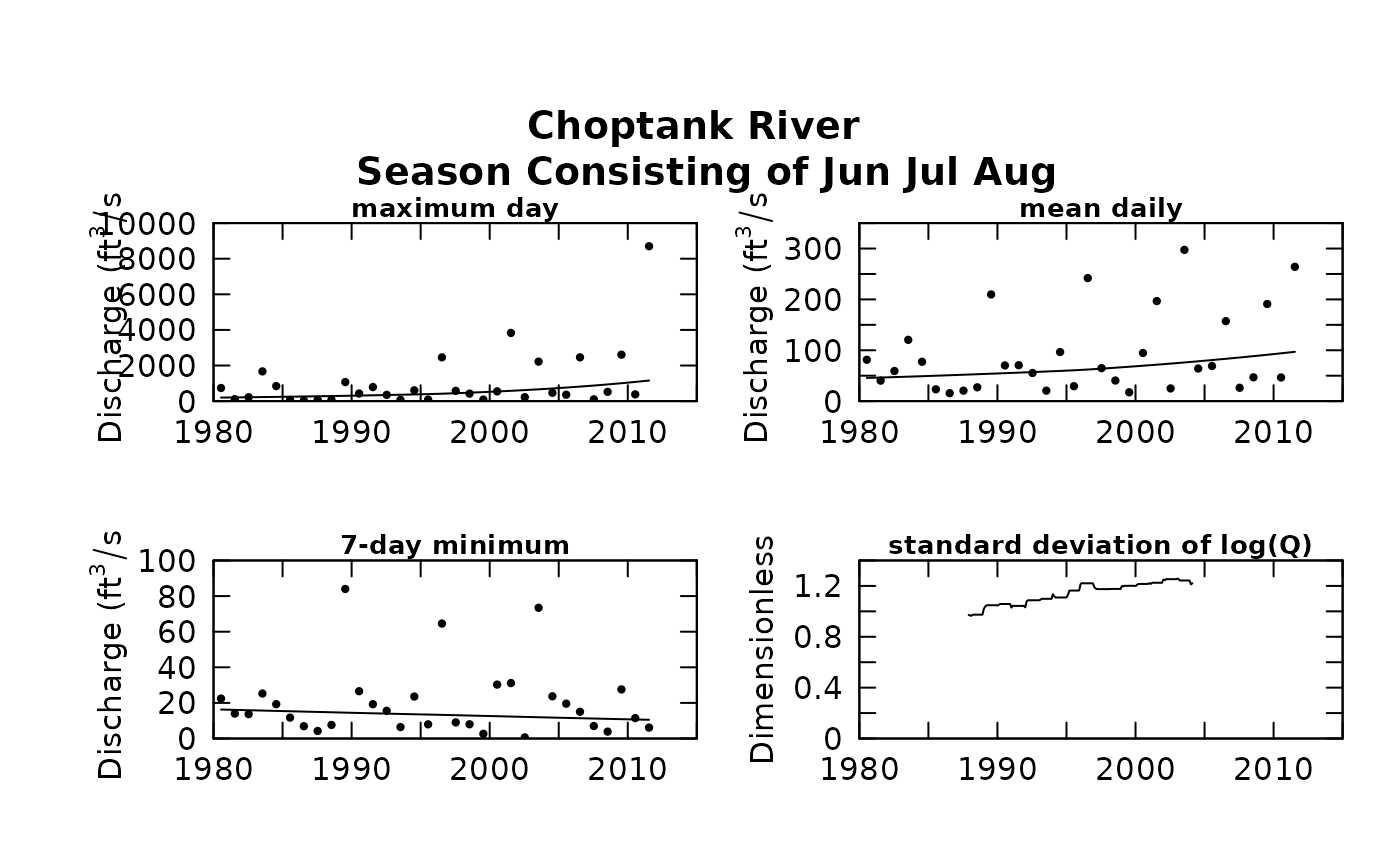Part of the flowHistory system. The four statistics are 1-day maximum, annual mean, annual 7-day minimum, and the running standard deviation of the log daily discharge values.

Although there are a lot of optional arguments to this function, most are set to a logical default.

Data come from named list, which contains a Daily dataframe with the daily flow data, and an INFO dataframe with metadata. Each graph shows a loess smooth of the data that are plotted.

## Usage

plotFour(eList, yearStart = NA, yearEnd = NA, printTitle = TRUE,
runoff = FALSE, qUnit = 1, window = 15, cex = 0.8, cex.axis = 1.2,
cex.main = 1.2, col = "black", lwd = 1, ...)

## Arguments

eList

named list with at least Daily and INFO dataframes

yearStart

A numeric value for year in which the graph should start, default is NA, which indicates that the graph should start with first annual value

yearEnd

A numeric value for year in which the graph should end, default is NA, which indicates that the graph should end with last annual value

printTitle

logical variable, if TRUE title is printed, if FALSE title is not printed, default is TRUE

runoff

logical variable, if TRUE the streamflow data are converted to runoff values in mm/day

qUnit

object of qUnit class printqUnitCheatSheet, or numeric represented the short code, or character representing the descriptive name.

window

numeric which is the full width, in years, of the time window over which the standard deviation is computed, default = 15

cex

numerical value giving the amount by which plotting symbols should be magnified

cex.axis

magnification to be used for axis annotation relative to the current setting of cex

cex.main

magnification to be used for main titles relative to the current setting of cex

col

color of points on plot, see ?par 'Color Specification'

lwd

number line width. Default is 1.

...

arbitrary graphical parameters that will be passed to genericEGRETDotPlot function (see ?par for options)

plotFlowSingle

## Examples

eList <- Choptank_eList
# \donttest{
#Water year:
plotFour(eList)# Graphs consisting of Jun-Aug
eList <- setPA(eList,paStart=6,paLong=3)
plotFour(eList)# }